# morse tutorial

#### 2019-02-14

The package morse is devoted to the analysis of data from standard toxicity tests. It provides a simple workflow to explore/visualize a data set, and compute estimations of risk assessment indicators. This document illustrates a typical use of morse on survival and reproduction data, which can be followed step-by-step to analyze new data sets.

# Survival data analysis at target time (TT)

The following example shows all the steps to perform survival analysis on standard toxicity test data and to produce estimated values of the $$LC_x$$. We will use a data set of the library named cadmium2, which contains both survival and reproduction data from a chronic laboratory toxicity test. In this experiment, snails were exposed to six concentrations of a metal contaminant (cadmium) during 56 days.

## Step 1: check the structure and the data set integrity

The data from a survival toxicity test should be gathered in a data.frame with a specific layout. This is documented in the paragraph on survData in the reference manual, and you can also inspect one of the data sets provided in the package (e.g., cadmium2). First, we load the data set and use the function survDataCheck() to check that it has the expected layout:

data(cadmium2)
survDataCheck(cadmium2)
## No message

The output ## No message just informs that the data set is well-formed.

## Step 2: create a survData object

The class survData corresponds to survival data and is the basic layout used for the subsequent operations. Note that if the call to survDataCheck() reports no error (i.e., ## No message), it is guaranteed that survData will not fail.

dat <- survData(cadmium2)
head(dat)
## # A tibble: 6 x 6
##    conc  time Nsurv Nrepro replicate Ninit
##   <dbl> <int> <int>  <int>     <dbl> <int>
## 1     0     0     5      0         1     5
## 2     0     3     5    262         1     5
## 3     0     7     5    343         1     5
## 4     0    10     5    459         1     5
## 5     0    14     5    328         1     5
## 6     0    17     5    742         1     5

## Step 3: visualize your data set

The function plot() can be used to plot the number of surviving individuals as a function of time for all concentrations and replicates.

plot(dat, pool.replicate = FALSE)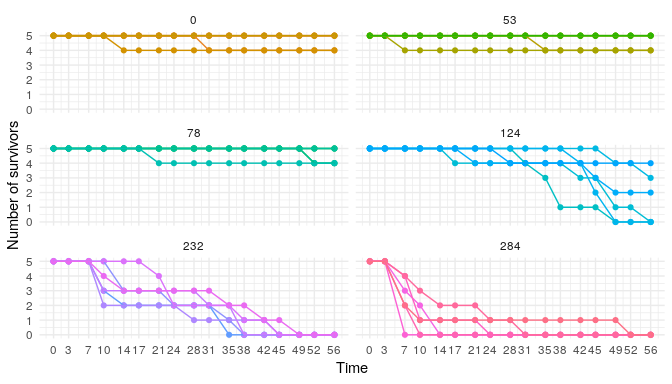Two graphical styles are available, "generic" for standard R plots or "ggplot" to call package ggplot2 (default). If argument pool.replicate is TRUE, datapoints at a given time-point and a given concentration are pooled and only the mean number of survivors is plotted. To observe the full data set, we set this option to FALSE.

By fixing the concentration at a (tested) value, we can visualize one subplot in particular:

plot(dat, concentration = 124, addlegend = TRUE,
pool.replicate = FALSE, style ="generic")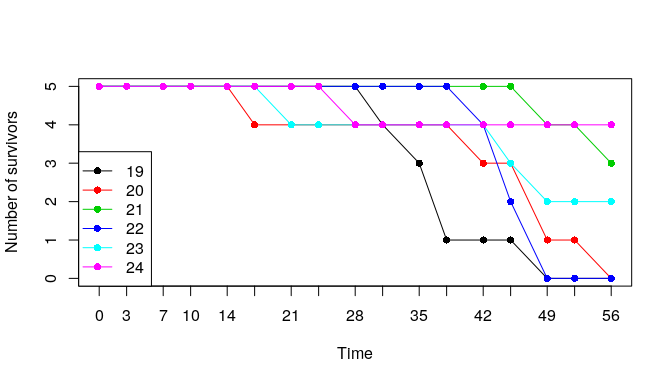We can also plot the survival rate, at a given time-point, as a function of concentration, with binomial confidence intervals around the data. This is achieved by using function plotDoseResponse() and by fixing the option target.time (default is the end of the experiment).

plotDoseResponse(dat, target.time = 21, addlegend = TRUE)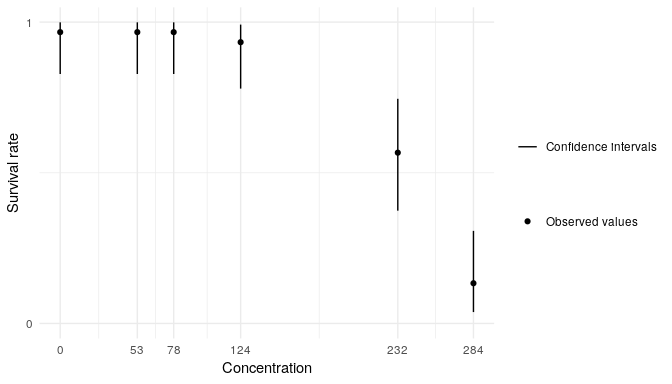Function summary() provides some descriptive statistics on the experimental design.

summary(dat)
##
## Number of replicates per time and concentration:
##      time
## conc  0 3 7 10 14 17 21 24 28 31 35 38 42 45 49 52 56
##   0   6 6 6  6  6  6  6  6  6  6  6  6  6  6  6  6  6
##   53  6 6 6  6  6  6  6  6  6  6  6  6  6  6  6  6  6
##   78  6 6 6  6  6  6  6  6  6  6  6  6  6  6  6  6  6
##   124 6 6 6  6  6  6  6  6  6  6  6  6  6  6  6  6  6
##   232 6 6 6  6  6  6  6  6  6  6  6  6  6  6  6  6  6
##   284 6 6 6  6  6  6  6  6  6  6  6  6  6  6  6  6  6
##
## Number of survivors (sum of replicates) per time and concentration:
##      0  3  7 10 14 17 21 24 28 31 35 38 42 45 49 52 56
## 0   30 30 30 30 29 29 29 29 29 28 28 28 28 28 28 28 28
## 53  30 30 29 29 29 29 29 29 29 29 28 28 28 28 28 28 28
## 78  30 30 30 30 30 30 29 29 29 29 29 29 29 29 29 27 27
## 124 30 30 30 30 30 29 28 28 27 26 25 23 21 18 11 11  9
## 232 30 30 30 22 18 18 17 14 13 12  8  4  3  1  0  0  0
## 284 30 30 15  7  4  4  4  2  2  1  1  1  1  1  1  0  0

## Step 4: fit an exposure-response model to the survival data at target time

Now we are ready to fit a probabilistic model to the survival data, in order to describe the relationship between the concentration in chemical compound and survival rate at the target time. Our model assumes this latter is a log-logistic function of the former, from which the package delivers estimates of the parameters. Once we have estimated the parameters, we can then calculate the $$LC_x$$ values for any $$x$$. All this work is performed by the survFitTT() function, which requires a survData object as input and the levels of $$LC_x$$ we want:

fit <- survFitTT(dat,
target.time = 21,
lcx = c(10, 20, 30, 40, 50))

The returned value is an object of class survFitTT providing the estimated parameters as a posterior1 distribution, which quantifies the uncertainty on their true value. For the parameters of the models, as well as for the $$LC_x$$ values, we report the median (as the point estimated value) and the 2.5 % and 97.5 % quantiles of the posterior (as a measure of uncertainty, a.k.a. credible intervals). They can be obtained by using the summary() method:

summary(fit)
## Summary:
##
## The  loglogisticbinom_3  model with a binomial stochastic part was used !
##
## Priors on parameters (quantiles):
##
##         50%      2.5%     97.5%
## b 1.000e+00 1.259e-02 7.943e+01
## d 5.000e-01 2.500e-02 9.750e-01
## e 1.227e+02 5.390e+01 2.793e+02
##
## Posteriors of the parameters (quantiles):
##
##         50%      2.5%     97.5%
## b 8.598e+00 3.986e+00 1.616e+01
## d 9.568e-01 9.102e-01 9.860e-01
## e 2.367e+02 2.105e+02 2.535e+02
##
## Posteriors of the LCx (quantiles):
##
##            50%      2.5%     97.5%
## LC10 1.833e+02 1.266e+02 2.138e+02
## LC20 2.012e+02 1.543e+02 2.259e+02
## LC30 2.143e+02 1.749e+02 2.349e+02
## LC40 2.257e+02 1.934e+02 2.435e+02
## LC50 2.367e+02 2.105e+02 2.535e+02

If the inference went well, it is expected that the difference between quantiles in the posterior will be reduced compared to the prior, meaning that the data were helpful to reduce the uncertainty on the true value of the parameters. This simple check can be performed using the summary function.

The fit can also be plotted:

plot(fit, log.scale = TRUE, adddata = TRUE,   addlegend = TRUE)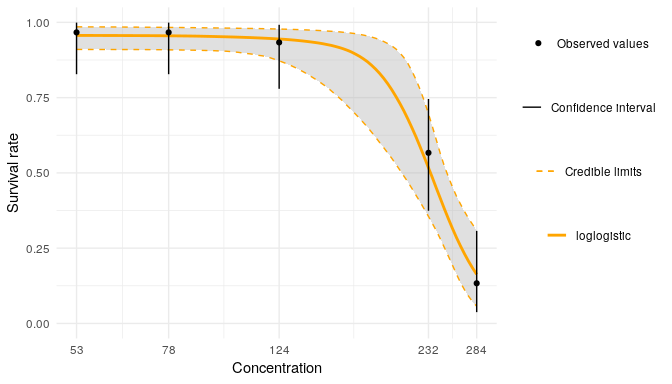This representation shows the estimated relationship between concentration of chemical compound and survival rate (orange curve). It is computed by choosing for each parameter the median value of its posterior. To assess the uncertainty on this estimation, we compute many such curves by sampling the parameters in the posterior distribution. This gives rise to the grey band, showing for any given concentration an interval (called credible interval) containing the survival rate 95% of the time in the posterior distribution. The experimental data points are represented in black and correspond to the observed survival rate when pooling all replicates. The black error bars correspond to a 95% confidence interval, which is another, more straightforward way to bound the most probable value of the survival rate for a tested concentration. In favorable situations, we expect that the credible interval around the estimated curve and the confidence interval around the experimental data largely overlap.

A similar plot is obtained with the style "generic":

plot(fit, log.scale = TRUE, style = "generic", adddata = TRUE, addlegend = TRUE)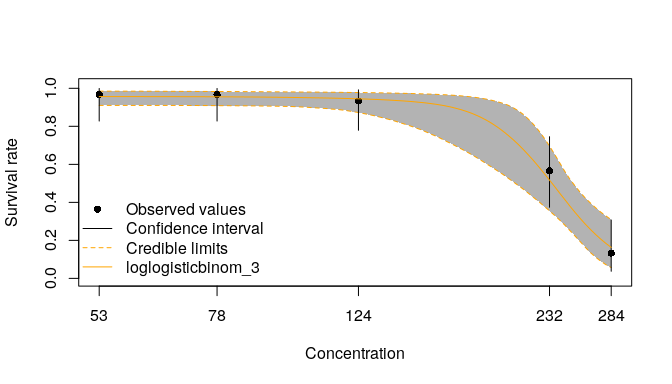Note that survFitTT() will warn you if the estimated $$LC_{x}$$ lie outside the range of tested concentrations, as in the following example:

data("cadmium1")
target.time = 21,
lcx = c(10, 20, 30, 40, 50))
## Warning: The LC50 estimation (model parameter e) lies outside the range of
## tested concentrations and may be unreliable as the prior distribution on
## this parameter is defined from this range !
plot(doubtful_fit, log.scale = TRUE, style = "ggplot", adddata = TRUE,
addlegend = TRUE)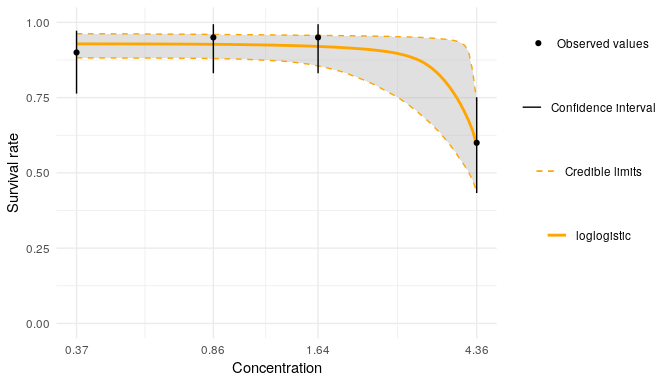In this example, the experimental design does not include sufficiently high concentrations, and we are missing measurements that would have a major influence on the final estimation. For this reason this result should be considered unreliable.

## Step 5: validate the model with a posterior predictive check

The fit can be further validated using so-called posterior predictive checks: the idea is to plot the observed values against the corresponding estimated predictions, along with their 95% credible interval. If the fit is correct, we expect to see 95% of the data inside the intervals.

ppc(fit)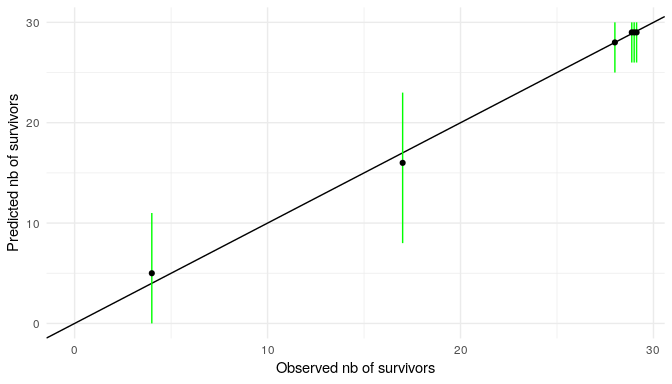In this plot, each black dot represents an observation made at a given concentration, and the corresponding number of survivors at target time is given by the value on the x-axis. Using the concentration and the fitted model, we can produce the corresponding prediction of the expected number of survivors at that concentration. This prediction is given by the y-axis. Ideally observations and predictions should coincide, so we’d expect to see the black dots on the points of coordinate $$Y = X$$. Our model provides a tolerable variation around the predited mean value as an interval where we expect 95% of the dots to be in average. The intervals are represented in green if they overlap with the line $$Y=X$$, and in red otherwise.

# Survival analysis using a toxicokinetic-toxicodynamic (TKTD) model: GUTS

The steps for a TKTD data analysis are absolutely analogous to what we described for the analysis at target time. Here the goal is to estimate the relationship between chemical compound concentration, time and survival rate using the GUTS models. GUTS, for General Unified Threshold models of Survival, is a TKTD models generalising most of existing mechanistic models for survival description. For details about GUTS models, see the vignette theory under modelling approaches, and the included references.

## GUTS model with constant exposure concentrations

Here is a typical session to analyse concentration-dependent time-course data using the so-called “Stochastic Death” (SD) model:

# (1) load data set
data(propiconazole)

# (2) check structure and integrity of the data set
survDataCheck(propiconazole)
## No message
# (3) create a survData object
dat <- survData(propiconazole)

# (4) represent the number of survivors as a function of time
plot(dat, pool.replicate = FALSE)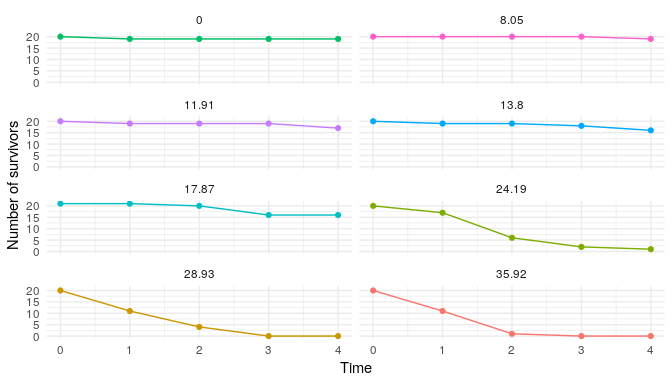# (5) check information on the experimental design
summary(dat)
##
## Number of replicates per time and concentration:
##        time
## conc    0 1 2 3 4
##   0     1 1 1 1 1
##   8.05  1 1 1 1 1
##   11.91 1 1 1 1 1
##   13.8  1 1 1 1 1
##   17.87 1 1 1 1 1
##   24.19 1 1 1 1 1
##   28.93 1 1 1 1 1
##   35.92 1 1 1 1 1
##
## Number of survivors (sum of replicates) per time and concentration:
##        0  1  2  3  4
## 0     20 19 19 19 19
## 8.05  20 20 20 20 19
## 11.91 20 19 19 19 17
## 13.8  20 19 19 18 16
## 17.87 21 21 20 16 16
## 24.19 20 17  6  2  1
## 28.93 20 11  4  0  0
## 35.92 20 11  1  0  0

### GUTS model “SD”

To fit the Stochastic Death model, we have to specify the model_type as "SD":

# (6) fit the TKTD model SD
fit_cstSD <- survFit(dat, quiet = TRUE, model_type = "SD")

Then, the summary() function provides parameters estimates as medians and 95% credible intervals.

# (7) summary of parameters estimates
summary(fit_cstSD)
## Summary:
##
## Priors of the parameters (quantiles) (select with '$Qpriors'): ## ## parameters median Q2.5 Q97.5 ## kd 4.157e-02 2.771e-04 6.236e+00 ## hb 1.317e-02 2.708e-04 6.403e-01 ## z 1.700e+01 8.171e+00 3.539e+01 ## kk 5.727e-03 1.021e-05 3.212e+00 ## ## Posteriors of the parameters (quantiles) (select with '$Qposteriors'):
##
##  parameters    median      Q2.5     Q97.5
##          kd 2.184e+00 1.581e+00 3.506e+00
##          hb 2.668e-02 1.274e-02 4.893e-02
##           z 1.689e+01 1.546e+01 1.866e+01
##          kk 1.231e-01 7.867e-02 1.894e-01
# OR
fit_cstSD$estim.par ## parameters median Q2.5 Q97.5 ## 1 kd 2.18384163 1.58096837 3.50550149 ## 2 hb 0.02668378 0.01274180 0.04892699 ## 3 z 16.88632674 15.45933339 18.66204545 ## 4 kk 0.12313328 0.07867125 0.18941270 Once fitting is done, we can compute posteriors vs. priors distribution with the function plot_prior_post() as follow: plot_prior_post(fit_cstSD)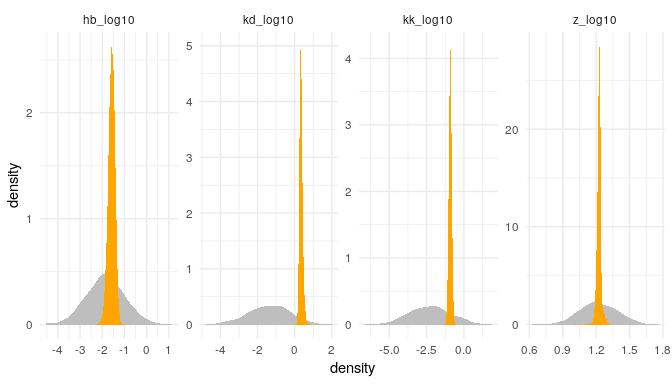The plot() function provides a representation of the fitting for each replicates plot(fit_cstSD)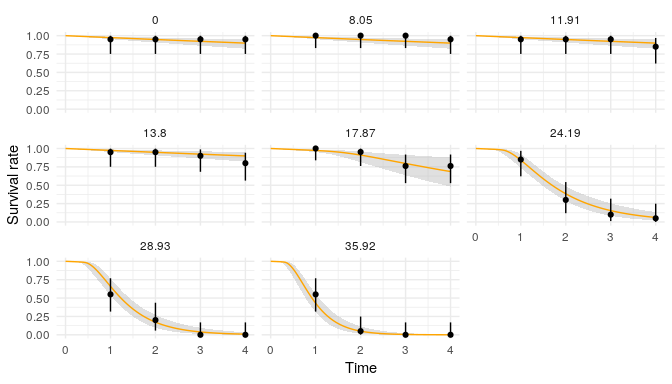Original data can be removed by using the option adddata = FALSE plot(fit_cstSD, adddata = FALSE)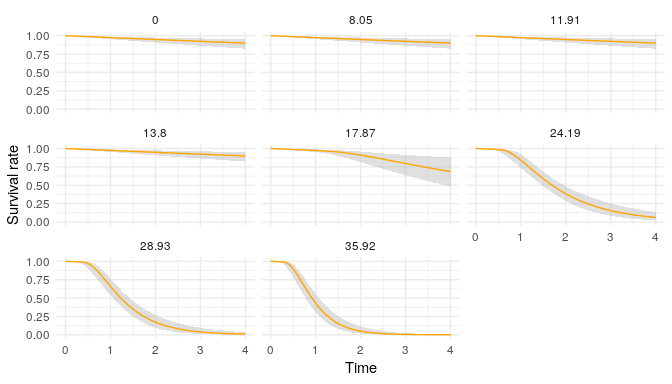A posterior predictive check is also possible using function ppc(): ppc(fit_cstSD)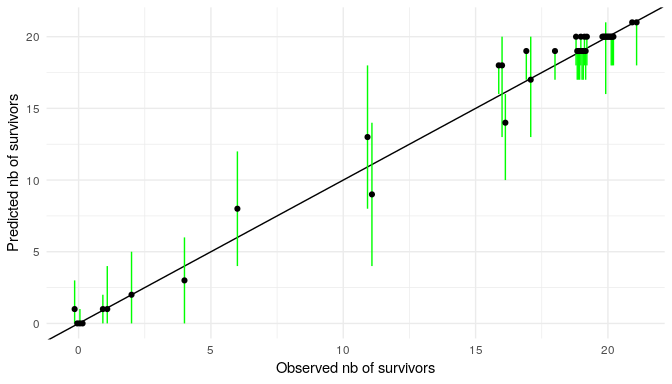### GUTS model “IT” The Individual Tolerance (IT) model is a variant of TKTD survival analysis. It can also be used with morse as demonstrated hereafter. For the IT model, we have to specify the model_type as "IT": fit_cstIT <- survFit(dat, quiet = TRUE, model_type = "IT") We can first get a summary of the estimated parameters: summary(fit_cstIT) ## Summary: ## ## Priors of the parameters (quantiles) (select with '$Qpriors'):
##
##  parameters    median      Q2.5     Q97.5
##          kd 4.157e-02 2.771e-04 6.236e+00
##          hb 1.317e-02 2.708e-04 6.403e-01
##       alpha 1.700e+01 8.171e+00 3.539e+01
##        beta 1.000e+00 1.259e-02 7.943e+01
##
## Posteriors of the parameters (quantiles) (select with '$Qposteriors'): ## ## parameters median Q2.5 Q97.5 ## kd 7.186e-01 5.289e-01 9.413e-01 ## hb 1.597e-02 3.682e-03 3.763e-02 ## alpha 1.771e+01 1.505e+01 2.026e+01 ## beta 6.685e+00 4.875e+00 9.103e+00 # OR fit_cstIT$estim.par
##   parameters     median         Q2.5       Q97.5
## 1         kd  0.7185555  0.528888116  0.94132495
## 2         hb  0.0159749  0.003681877  0.03763295
## 3      alpha 17.7068809 15.046087432 20.25596345
## 4       beta  6.6854653  4.875476050  9.10324854

And the plot of posteriors vs. priors distributions:

plot_prior_post(fit_cstIT)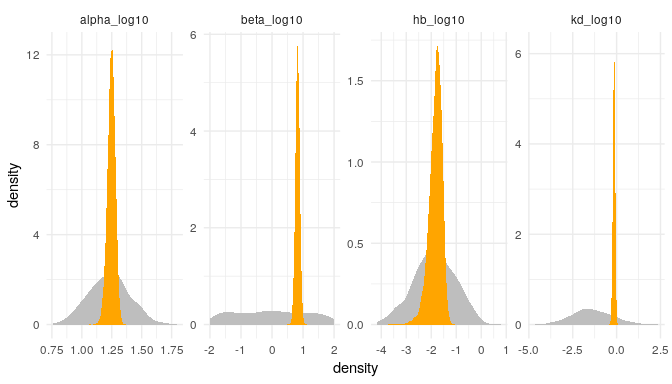plot(fit_cstIT)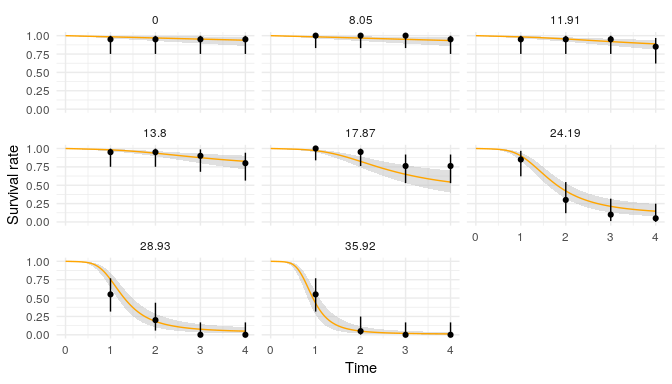ppc(fit_cstIT)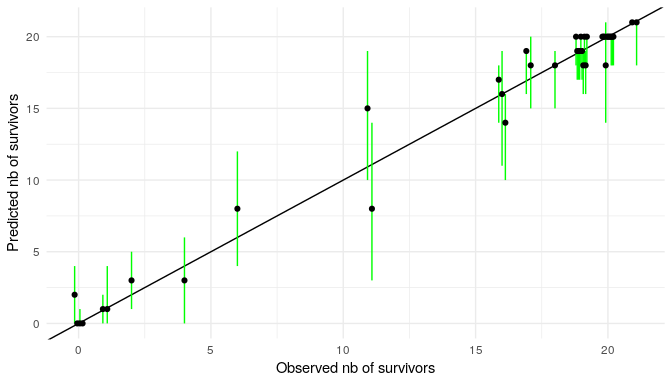## GUTS model under time-variable exposure concentration

Here is a typical session fitting an SD or an IT model for a data set under time-variable exposure scenario.

# (1) load data set
data("propiconazole_pulse_exposure")

# (2) check structure and integrity of the data set
survDataCheck(propiconazole_pulse_exposure)
## No message
# (3) create a survData object
dat <- survData(propiconazole_pulse_exposure)

# (4) represent the number of survivor as a function of time
plot(dat)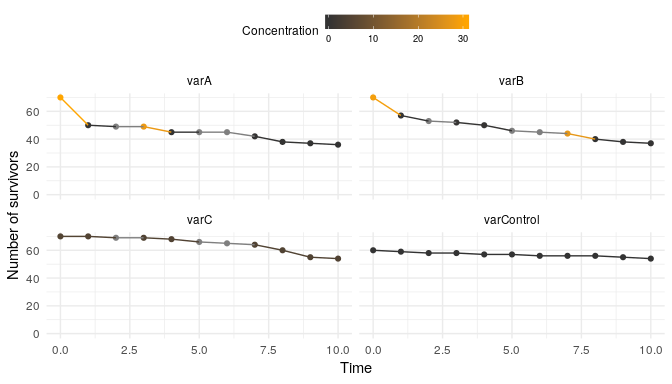# (5) check information on the experimental design
summary(dat)
##
## Occurence of 'replicate' for each 'time':
##             time
## replicate    0 0.96 1 1.96 2 2.96 3 3.96 4 4.96 4.97 5 5.96 6 6.96 7 7.96
##   varA       1    1 1    1 1    1 1    1 1    1    1 1    1 1    1 1    1
##   varB       1    1 1    1 1    1 1    1 1    1    1 1    1 1    1 1    1
##   varC       1    1 1    1 1    1 1    1 1    1    1 1    1 1    1 1    1
##   varControl 1    0 1    0 1    0 1    0 1    0    0 1    0 1    0 1    0
##             time
## replicate    8 9 9.96 10
##   varA       1 1    1  1
##   varB       1 1    1  1
##   varC       1 1    1  1
##   varControl 1 1    0  1
##
## Number of survivors per 'time' and 'replicate':
##             0 0.96  1 1.96  2 2.96  3 3.96  4 4.96 4.97  5 5.96  6 6.96  7
## varA       70   NA 50   NA 49   NA 49   NA 45   NA   NA 45   NA 45   NA 42
## varB       70   NA 57   NA 53   NA 52   NA 50   NA   NA 46   NA 45   NA 44
## varC       70   NA 70   NA 69   NA 69   NA 68   NA   NA 66   NA 65   NA 64
## varControl 60   NA 59   NA 58   NA 58   NA 57   NA   NA 57   NA 56   NA 56
##            7.96  8  9 9.96 10
## varA         NA 38 37   NA 36
## varB         NA 40 38   NA 37
## varC         NA 60 55   NA 54
## varControl   NA 56 55   NA 54
##
## Concentrations per 'time' and 'replicate':
##                0  0.96    1 1.96  2 2.96     3  3.96    4 4.96 4.97  5
## varA       30.56 27.93 0.00 0.26 NA 0.21 27.69 26.49 0.00 0.18 0.18 NA
## varB       28.98 27.66 0.00 0.27 NA 0.26  0.26  0.26 0.26 0.25 0.25 NA
## varC        4.93  4.69 4.69 4.58 NA 4.58  4.58  4.54 4.54 4.58 4.71 NA
## varControl  0.00    NA 0.00   NA  0   NA  0.00    NA 0.00   NA   NA  0
##            5.96  6 6.96     7  7.96    8    9 9.96   10
## varA       0.18 NA 0.14  0.14  0.18 0.18 0.00 0.00 0.00
## varB       0.03 NA 0.00 26.98 26.28 0.00 0.12 0.12 0.12
## varC       4.71 NA 4.60  4.60  4.59 4.59 4.46 4.51 4.51
## varControl   NA  0   NA  0.00    NA 0.00 0.00   NA 0.00

### GUTS model “SD”

# (6) fit the TKTD model SD
fit_varSD <- survFit(dat, quiet = TRUE, model_type = "SD")
## Warning: The estimation of the dominant rate constant (model parameter kd) lies
##     outside the range used to define its prior distribution which indicates that this
##     rate is very high and difficult to estimate from this experiment !
## Warning: The estimation of Non Effect Concentration threshold (NEC)
##       (model parameter z) lies outside the range of tested concentration
##       and may be unreliable as the prior distribution on this parameter is
##       defined from this range !
# (7) summary of the fit object
summary(fit_varSD)
## Summary:
##
## Priors of the parameters (quantiles) (select with '$Qpriors'): ## ## parameters median Q2.5 Q97.5 ## kd 2.683e-02 1.119e-04 6.434e+00 ## hb 8.499e-03 1.094e-04 6.606e-01 ## z 9.154e-01 3.212e-02 2.608e+01 ## kk 5.080e-02 4.342e-06 5.942e+02 ## ## Posteriors of the parameters (quantiles) (select with '$Qposteriors'):
##
##  parameters    median      Q2.5     Q97.5
##          kd 3.693e+00 1.567e+00 2.634e+01
##          hb 2.320e-02 1.638e-02 3.110e-02
##           z 2.097e+01 2.626e+00 3.181e+01
##          kk 8.648e-02 5.431e-03 7.014e-01
plot_prior_post(fit_varSD)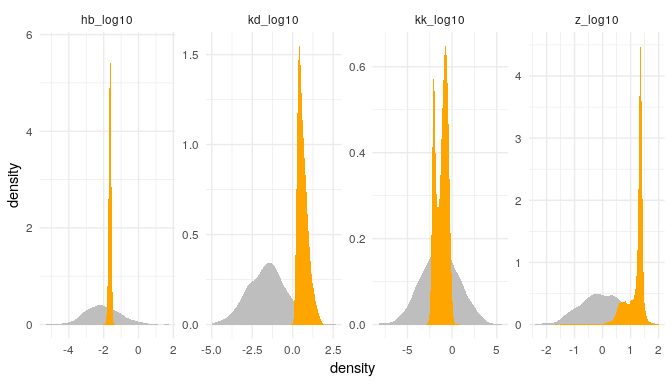plot(fit_varSD)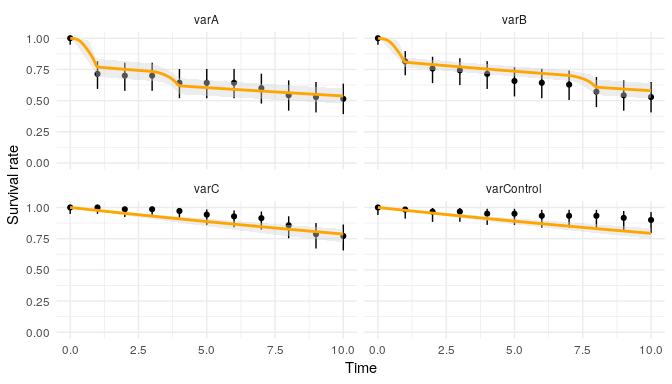ppc(fit_varSD)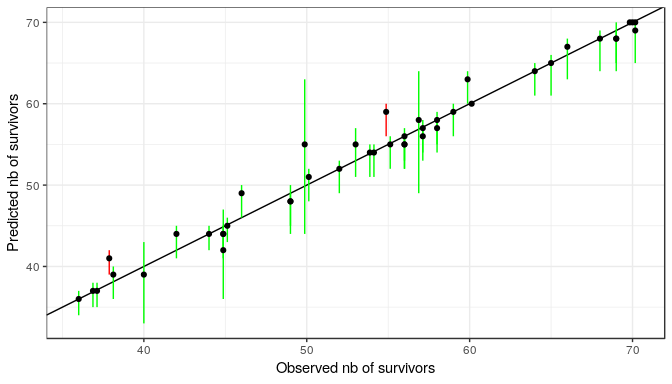### GUTS model “IT”

# fit a TKTD model IT
fit_varIT <- survFit(dat, quiet = TRUE, model_type = "IT")
## Warning: The estimation of the dominant rate constant (model parameter kd) lies
##     outside the range used to define its prior distribution which indicates that this
##     rate is very high and difficult to estimate from this experiment !
## Warning: The estimation of log-logistic median (model parameter alpha)
##       lies outside the range of tested concentration and may be unreliable as
##       the prior distribution on this parameter is defined from this range !
# (7) summary of the fit object
summary(fit_varIT)
## Summary:
##
## Priors of the parameters (quantiles) (select with '$Qpriors'): ## ## parameters median Q2.5 Q97.5 ## kd 2.683e-02 1.119e-04 6.434e+00 ## hb 8.499e-03 1.094e-04 6.606e-01 ## alpha 9.154e-01 3.212e-02 2.608e+01 ## beta 1.000e+00 1.259e-02 7.943e+01 ## ## Posteriors of the parameters (quantiles) (select with '$Qposteriors'):
##
##  parameters    median      Q2.5     Q97.5
##          kd 4.301e-01 5.587e-04 1.842e+01
##          hb 2.430e-02 1.619e-02 3.327e-02
##       alpha 1.765e+01 2.042e-01 3.943e+01
##        beta 2.236e+00 4.916e-01 2.359e+01
plot_prior_post(fit_varIT)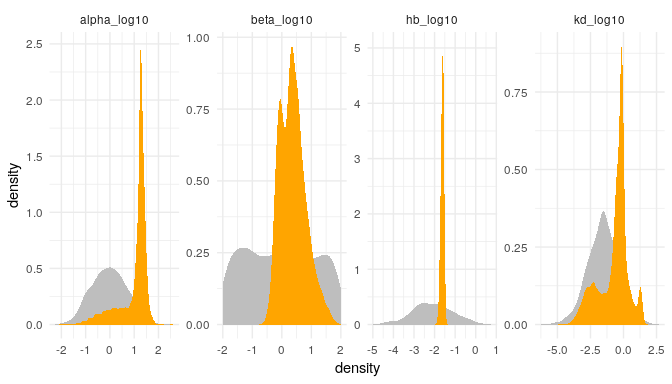plot(fit_varIT)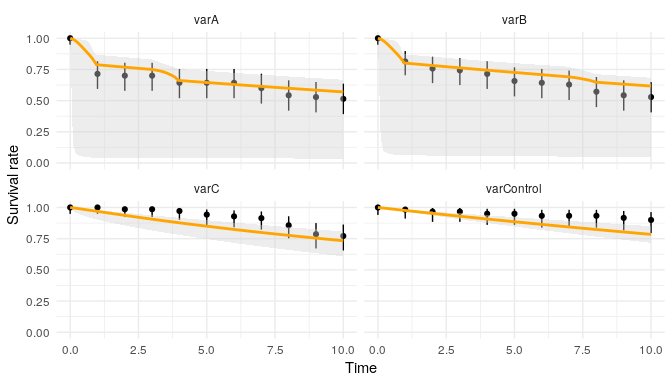ppc(fit_varIT)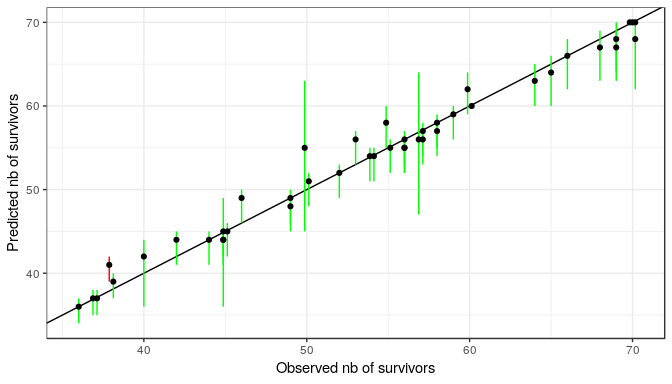## Computing prediction

GUTS models can be used to simulate the survival of the organisms under any exposure pattern, using the calibration done with function survFit() from observed data. The function for prediction is called predict() and returns an object of class survFitPredict.

# (1) upload or build a data frame with the exposure profile
# argument replicate is used to provide several profiles of exposure
data_4prediction <- data.frame(time = c(1:10, 1:10),
conc = c(c(0,0,40,0,0,0,40,0,0,0),
c(21,19,18,23,20,14,25,8,13,5)),
replicate = c(rep("pulse", 10), rep("random", 10)))

# (2) Use the fit on constant exposure propiconazole with model SD (see previously)
predict_PRZ_cstSD_4pred <- predict(object = fit_cstSD, data_predict = data_4prediction)

From an object survFitPredict, results can ben plotted with function plot():

# (3) Plot the predicted survival rate under the new exposure profiles.
plot(predict_PRZ_cstSD_4pred)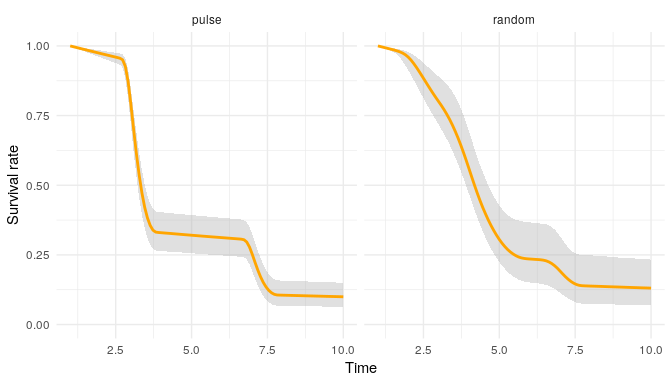### Robust ODE solver with deSolve

It appears that with some extreme data set, the fast way used to compute predictions return NA data, due to numerical error (e.g. number greater or lower than $$10^{300}$$ or $$10^{-300}$$).

When this issue happens, the function predict() returns an error, with the message providing the way to use the robust implementation with ODE solver provided by deSolve.

This way is implemented through the use of the function predict_ode(). Robustness goes often with longer time to compute. Time to compute can be long, so we use by default MCMC chain size of 1000 independent iterations.

predict_PRZ_cstSD_4pred_ode <- predict_ode(object = fit_cstSD, data_predict = data_4prediction)

This new object predict_PRZ_cstSD_4pred_ode is a survFitPredict object and so it has exactly the same properties as an object returned by a predict() function.

Note that since predict_ode() can be very long to compute, the mcmc_size is reduced to 1000 MCMC chains by default.

See for instance, with the plot:

plot(predict_PRZ_cstSD_4pred_ode)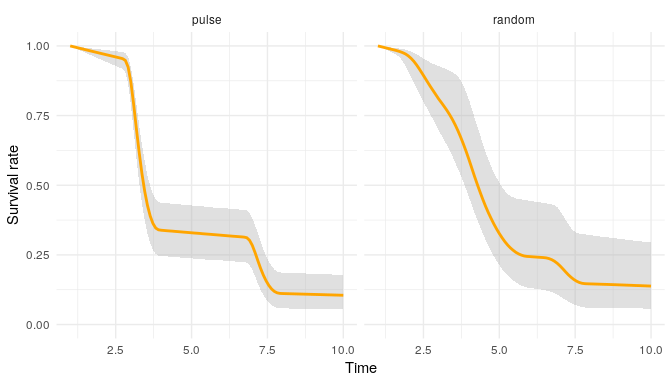### Removing background mortality in predictions

While the model has been estimated using the background mortality parameter hb, it can be interesting to see the prediction without it. This is possible with the argument hb_value. If TRUE, the background mortality is taken into account, and if FALSE, the background mortality is set to $$0$$ in the prediction.

# Use the same data set profile to predict without 'hb'
predict_PRZ_cstSD_4pred_hbOUT <- predict(object = fit_cstSD, data_predict = data_4prediction, hb_value = FALSE)
# Plot the prediction:
plot(predict_PRZ_cstSD_4pred_hbOUT)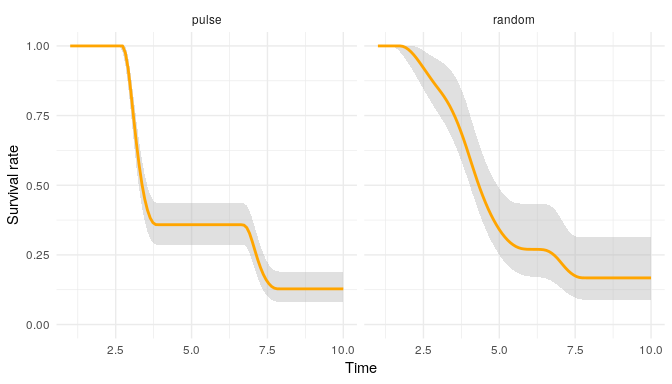## Validation criteria: EFSA recommendations

Following EFSA recommendations, the next functions compute qualitative and quantitative model performance criteria suitable for GUTS, and TKTD modelling in general: the percentage of observations within the 95% credible interval of the Posterior Prediction Check (PPC), the Normalised Root Mean Square Error (NRMSE) and the Survival-Probability Prediction Error (SPPE).

PPC

The PPC compares the predicted median numbers of survivors associated to their uncertainty limits with the observed numbers of survivors. This can be visualised by plotting the predicted versus the observed values and counting how frequently the confidence/credible limits intersect with the 1:1 prediction line [see previous plot]. Based on experience, PPC resulting in less than 50% of the observations within the uncertainty limits indicate poor model performance.

Normalised Root Mean Square Error NRMSE

NRMSE criterion is also based on the expectation that predicted and observed survival numbers matches the 1:1 line in a scatter plot. The criterion is based on the classical root-mean-square error (RMSE), used to aggregate the magnitudes of the errors in predictions for various time-points into a single measure of predictive power. In order to provide a criterion expressed as a percentage, it is suggested using a normalised RMSE by the mean of the observations.

$NRMSE = \frac{RMSE}{\overline{Y}} = \frac{1}{\overline{Y}} \sqrt{\frac{1}{n} \sum_{i=1}^{n} (Y_{obs,i} - Y_{pred,i})^2} \times 100$

Survival Probability Prediction Error (SPPE)

The SPPE indicator is negative (between 0 and -100%) for an underestimation of effects, and positive (between 0 and 100%) for an overestimation of effects. An SPPE value of 0% means an exact prediction of the observed survival probability at the end of the experiment.

$SPPE = \left( \frac{Y_{obs, t_{end}}}{Y_{init}} - \frac{Y_{pred, t_{end}}}{Y_{init}} \right) \times 100 = \frac{Y_{obs, t_{end}} - Y_{pred, t_{end}}}{Y_{init}} \times 100$

### Computing predictions with number of survivors

For NRMSE and SPPE, we need to compute the number of survivors. To do so, we use the function predict_Nsurv() where two arguments are required: the first argument is a survFit object, and the other is a data set with four columns (time, conc, replicate and Nsurv). Contrary to the function predict(), here the column Nsurv is necessary.

predict_Nsurv_PRZ_SD_cstTOcst <- predict_Nsurv(fit_cstSD, propiconazole)
## Note that computing can be quite long (several minutes).
predict_Nsurv_PRZ_SD_varTOcst <- predict_Nsurv(fit_varSD, propiconazole)
## Note that computing can be quite long (several minutes).
predict_Nsurv_PRZ_SD_cstTOvar <- predict_Nsurv(fit_cstSD, propiconazole_pulse_exposure)
## Note that computing can be quite long (several minutes).
predict_Nsurv_PRZ_SD_varTOvar <- predict_Nsurv(fit_varSD, propiconazole_pulse_exposure)
## Note that computing can be quite long (several minutes).

### Robust implementation with ODE solver predict_Nsurv_ode

For the same reason that a predict_ode function as been implemented to compute predict function using the ODE solver of deSolve, a predict_Nsurv_ode function as been implemented as equivalent to predict_Nsurv. The time to compute is subtentially longer than the original function.

predict_Nsurv_PRZ_SD_cstTOcst_ode <- predict_Nsurv_ode(fit_cstSD, propiconazole)
## Note that computing can be quite long (several minutes).

When both function work well, their results are identical (or highly similar):

plot(predict_Nsurv_PRZ_SD_cstTOcst)
plot(predict_Nsurv_PRZ_SD_cstTOcst_ode)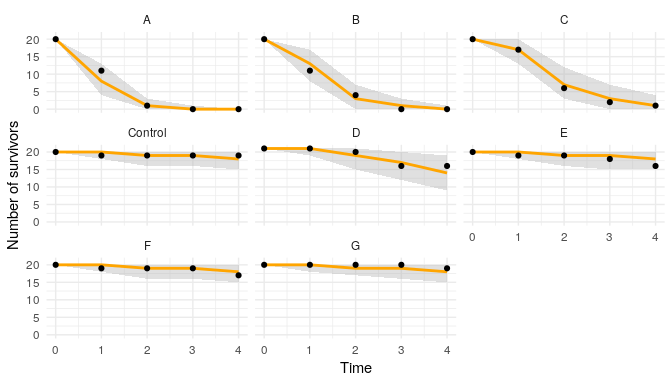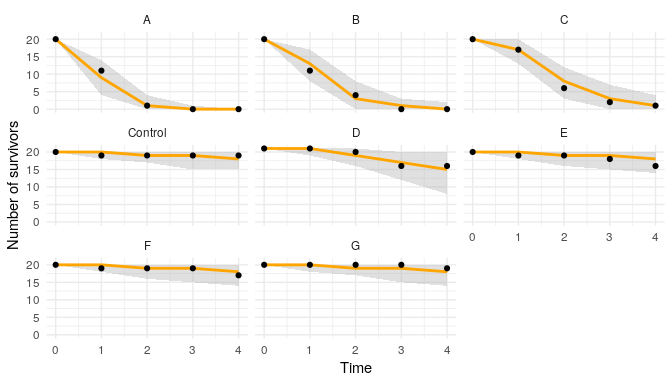plot(predict_Nsurv_PRZ_SD_cstTOcst_ode)